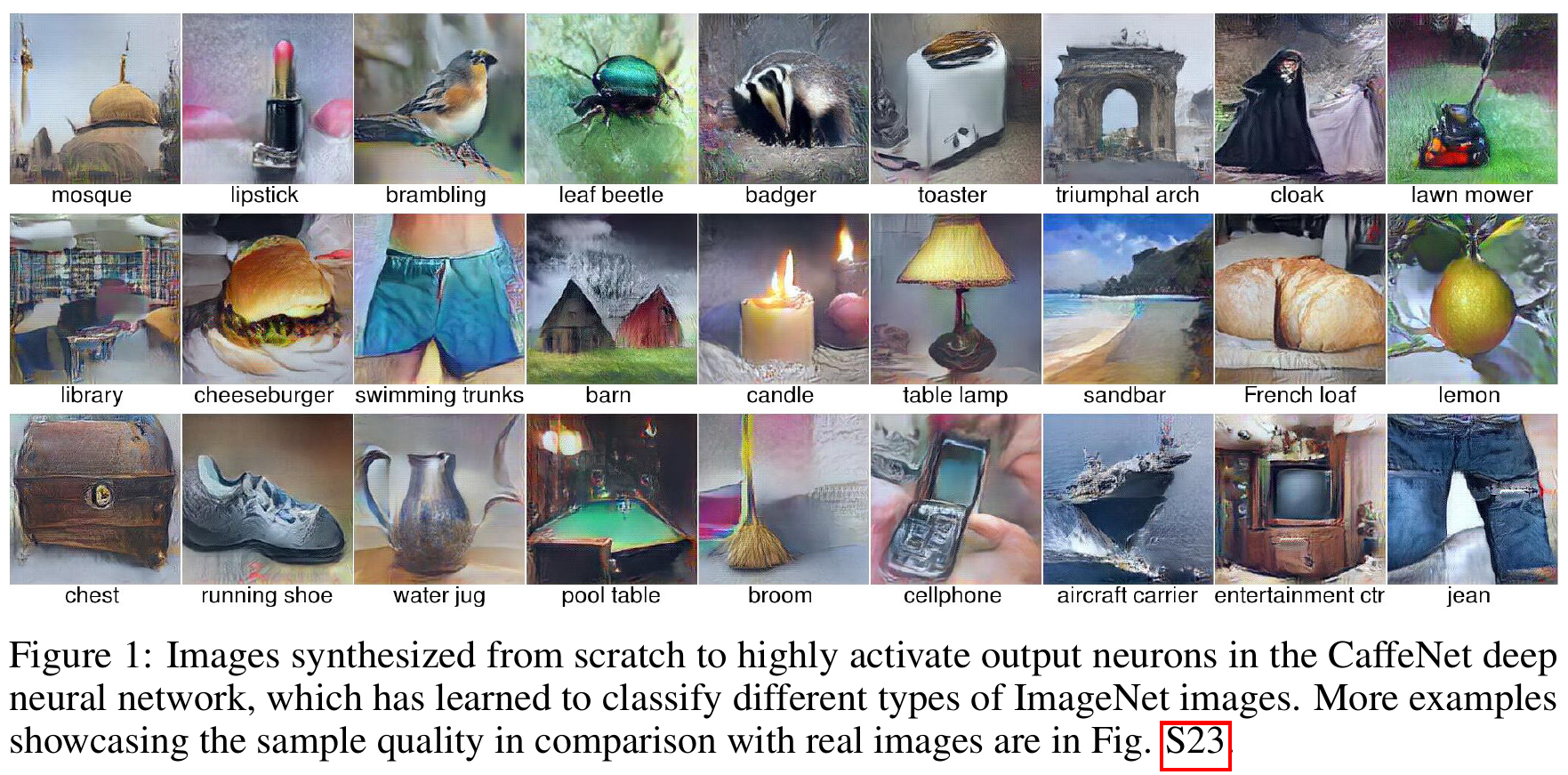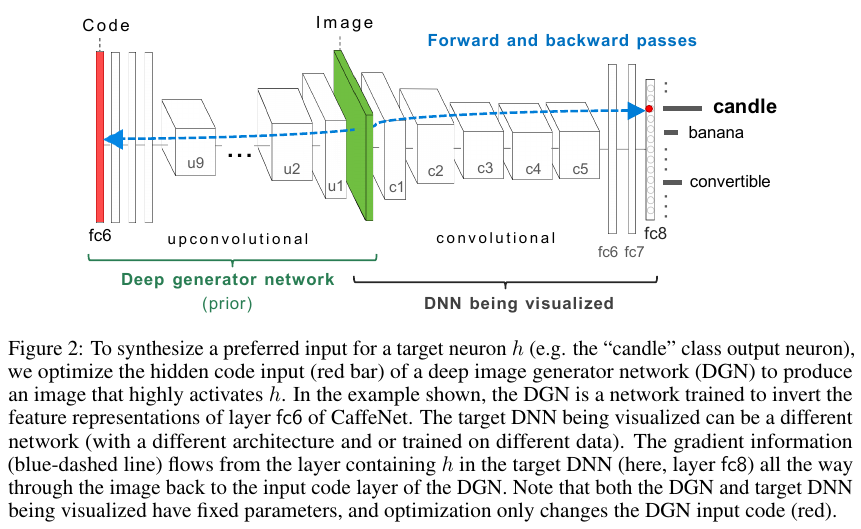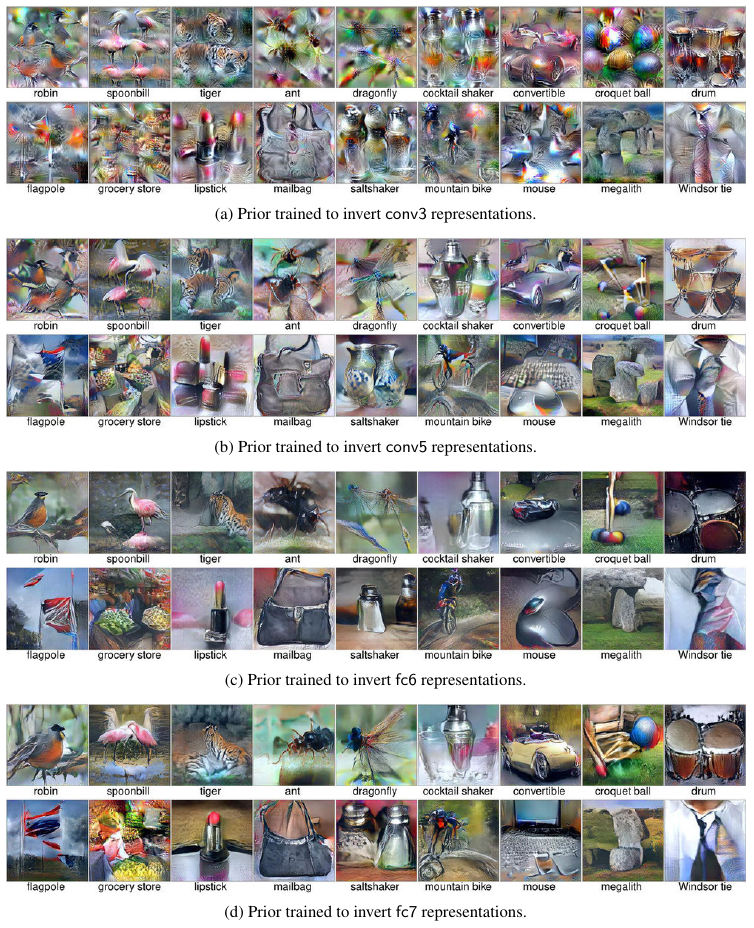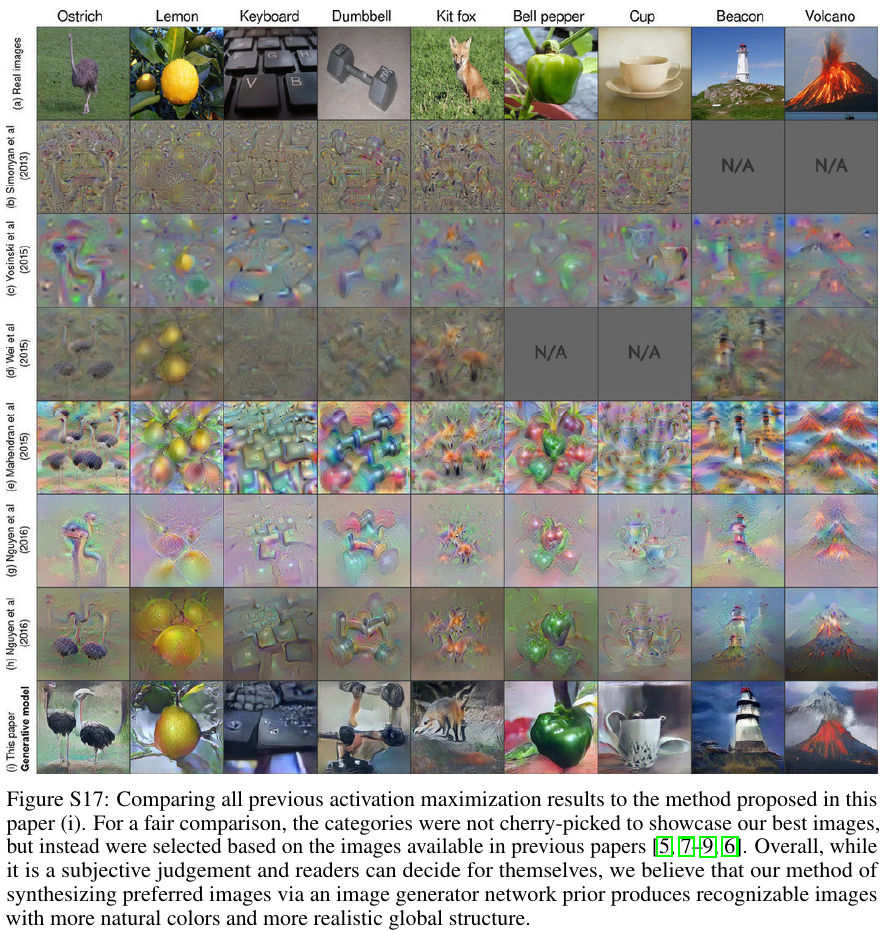This paper introduces DGN-AM: Deep Generator Network for Activation Maximization.# Training process

The training process involves 4 convolutional networks:

1. $$E$$, a fixed encoder network (the network being visualized)
2. $$G$$, a generator network that should be able to recover the original image from the output of $$E$$
3. $$C$$, a fixed “comparator” network
4. $$D$$, a discriminator

$$G$$ is trained to invert a feature representation extracted by $$E$$, and has to satisfy 3 objectives:

1. For a feature vector $$y = E(x)$$, the sythesized image $$G(y)$$ has to be close to the original image $$x$$
2. The features of the sythesized image $$C(G(y))$$ have to be close to those of the real image $$C(x)$$
3. $$D$$ should be unable to distinguish $$G(y)$$ from real images (like a GAN)

Architectures of the networks:

• $$E$$ is CaffeNet (pretty much AlexNet) truncated at a certain layer
• $$C$$ is CaffeNet up to layer pool5 (the last pooling layer before the first FC)
• $$D$$ is a convolutional network with 5 conv + 2 FC
• $$G$$ is an “upconvolutional” architecture with 9 upconv + 3 FC

# Choice of layer for representation

The best layer was determined empirically to be fc6.# Comparison with previous work# Applications

• Generate images that maximally activate a class neuron
• Generate images that maximally activate a hidden neuron
• Watch how features evolve during training
• “Produce creative, original art by synthesizing images that activate two neurons at the same time.” (See images in section S8.)